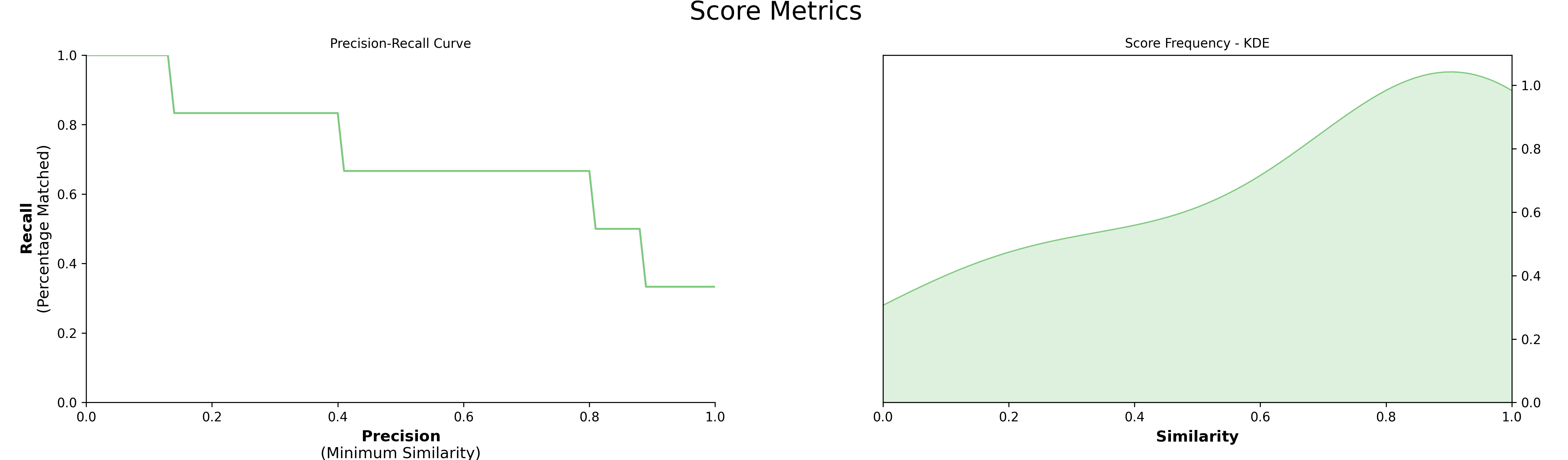# Custom Models¶

Although PolyFuzz has several models implemented, what if you have developed your own? What if you want a different similarity/distance measure that is not defined in PolyFuzz? That is where custom models come in. If you follow the structure of PolyFuzz's `BaseMatcher` you can quickly implement any model you would like.

You simply create a class using `BaseMatcher`, make sure it has a function `match` that inputs two lists and outputs a pandas dataframe. That's it!

We start by creating our own model that implements the ratio similarity measure from RapidFuzz:

``````import numpy as np
import pandas as pd
from rapidfuzz import fuzz

from polyfuzz import PolyFuzz
from polyfuzz.models import BaseMatcher

class MyModel(BaseMatcher):
def match(self, from_list, to_list, **kwargs):
# Calculate distances
matches = [[fuzz.ratio(from_string, to_string) / 100
for to_string in to_list] for from_string in from_list]

# Get best matches
mappings = [to_list[index] for index in np.argmax(matches, axis=1)]
scores = np.max(matches, axis=1)

# Prepare dataframe
matches = pd.DataFrame({'From': from_list,
'To': mappings,
'Similarity': scores})
return matches
``````

`MyModel` can now be used within `PolyFuzz` and runs like every other model:

``````from_list = ["apple", "apples", "appl", "recal", "house", "similarity"]
to_list = ["apple", "apples", "mouse"]

custom_matcher = MyModel()

model = PolyFuzz(custom_matcher).match(from_list, to_list)
``````

Now we can visualize the results:

``````model.visualize_precision_recall(kde=True)
``````## fit, transform, fit_transform¶

Although the above model can be used in production using `fit`, it does not track its state between `fit` and `transform`. This is not necessary here, since edit distances should be recalculated but if you have embeddings that you do not want to re-calculate, then it is helpful to track the states between `fit` and `transform` so that embeddings do not need to be re-calculated. To do so, we can use the `re_train` parameter to define what happens if we re-train a model (for example when using `fit`) and what happens when we do not re-train a model (for example when using `transform`).

In the example below, when we set `re_train=True` we calculate the embeddings from both the `from_list` and `to_list` if they are defined and save the embeddings to the `self.embeddings_to` variable. Then, when we set `re_train=True`, we can prevent redoing the `fit` by leveraging the pre-calculated `self.embeddings_to` variable.

``````import numpy as np
from sentence_transformers import SentenceTransformer

from ._utils import cosine_similarity
from ._base import BaseMatcher

class SentenceEmbeddings(BaseMatcher):
def __init__(self, model_id):
super().__init__(model_id)
self.type = "Embeddings"

self.embedding_model = SentenceTransformer("all-MiniLM-L6-v2")
self.embeddings_to = None

def match(self, from_list, to_list, re_train=True) -> pd.DataFrame:
# Extract embeddings from the `from_list`
embeddings_from = self.embedding_model.encode(from_list, show_progress_bar=False)

# Extract embeddings from the `to_list` if it exists
if not isinstance(embeddings_to, np.ndarray):
if not re_train:
embeddings_to = self.embeddings_to
elif to_list is None:
embeddings_to = self.embedding_model.encode(from_list, show_progress_bar=False)
else:
embeddings_to = self.embedding_model.encode(to_list, show_progress_bar=False)

# Extract matches
matches = cosine_similarity(embeddings_from, embeddings_to, from_list, to_list)

self.embeddings_to = embeddings_to

return matches
``````

Then, we can use it as follows:

``````from_list = ["apple", "apples", "appl", "recal", "house", "similarity"]
to_list = ["apple", "apples", "mouse"]

custom_matcher = MyModel()

model = PolyFuzz(custom_matcher).fit(from_list)
``````

By using the `.fit` function, embeddings are created from the `from_list` variable and saved. Then, when we run `model.transform(to_list)`, the embeddings created from the `from_list` variable do not need to be recalculated.